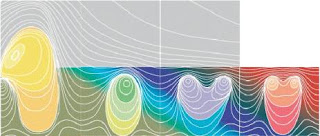## No More Secrets II

Bernhard Riemann wrote only one 8 page paper in number theory . It listed a number of conjectures, the most famous naturally being the Hypothesis. Let $\pi (N)$ be the number of primes less than or equal to $N$. Also recall the function

Li$(x) = \int_2^x \frac{dt}{\textrm{log} t}$

Another conjecture (later proved by von Mangoldt) that Riemann stated in his paper was that there should be a formula for $\pi (x)$ – Li$(x)$ valid for any $x > 1$. The only tricky term in the formula is a sum over the complex zeros $\rho$ of the $\zeta$ function,

$\sum_{\rho} \frac{x^{\rho}}{\rho}$

So the zeroes encode precise information about the distribution of the prime numbers. This imbues these points of the complex plane with a special significance, just as the ordinals along the real axis are also special because they represent ordinals, which we know are about counting elements of sets, or Euler characteristics for categories. Maybe the other numbers in the complex plane should be viewed this way as well. In other words, the complex plane is not given a priori as a boring set obeying the axioms of a field.

A topos theorist cannot escape such ideas, because number fields become heinously complicated. The ordinals make sense enough. Categories such as Set have an object of Natural Numbers. From this one can build the integers and the rationals, but then one has to worry about what one means by the real numbers because the set theoretic definitions don’t all necessarily agree in other toposes. And why should we care more about the reals, from the prime at infinity, than the p-adics? The p-adic number fields can fit into the complex plane via Chistyakov’s beautiful fractal patterns. From du Sautoy we also have some beautiful images of the $\zeta$ function:H. Davenport, Multiplicative Number Theory (LNM 74)

## 5 Responses so far »

1. 1### Mahndisa S. Rigmaiden said,

02 17 07

Hey Kea:
Good post. Fractals, scaling transformations and everything are all related to p-adics! The connexions are becoming richer each moment. I just found generating series for the reciprocal powers of Mersenne numbers in binary. Pretty cool stuff because the relations apply for any expansion of that type.

I will do a post expounding upon some of the ideas from the previous post. Suffice it to say, Reimann was clever, but Charles Pierce took those thoughts to another level…(perhaps without knowing it!)

2. 2### Kea said,

Mahndisa, you’re leaping ahead of all of us here! Personally I haven’t managed to make any direct links to Peirce yet, but you have the right idea for sure: numbers through diagrammatic reasoning. Peirce was way ahead of his time, so he won’t have realised how difficult the issues with set theory would become in the 20th century.

3. 3### a quantum diaries survivor said,

Hi Marni (or do you prefer Kea?),

fascinating! You would be surprised to see how little I know about the Riemann zeta function…

One thing which bothers me though, is the latex in your posts supposed to show ? Is it my browser’s fault (of course it is, it’s IE) that I don’t see the formula in a readable way ?

Cheers,
T.

4. 4### Kea said,

Hi Tommaso

The latex is not ideal on anything other than Firefox – nothing we can do about that at this point, I’m afraid. I’m always Marni in everyday life – Kea was initially a forum username from the brief time when I was anonymous.

5. 5### Kea said,

You would be surprised to see how little I know about the Riemann zeta function…

Oh, but you know a LOT about the zeta function, Tommaso, because you are a particle physicist. You just don’t know it yet!